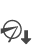About EasyScript
EasyScript is an expression syntax similar to the syntax used in Excel formulas. Like Excel, EasyScript provides functions for performing calculations on report data. In BIRT Designer Professional, EasyScript is supported in most places an expression is required. For example, when specifying an expression for a computed column, a column binding, a filter condition, or a map rule, you can use either JavaScript or EasyScript.
Choosing between EasyScript and JavaScript
You can use both JavaScript and EasyScript expressions in a report. For simple expressions or common calculations, the choice is often based on syntax familiarity or simplicity. Users who work with Excel functions will find EasyScript syntax familiar and easy to use.
The following example is an EasyScript expression that rounds values in a Price field to the nearest integer:
ROUND([Price])
The following example is the equivalent JavaScript expression:
Math.round(row["Price"])
Both expressions are straightforward, although one could argue that the EasyScript syntax is simpler. Now, compare the expressions used to round the Price values to 2 decimal places. In the following expressions, the first shows EasyScript syntax, and the second shows JavaScript syntax:
ROUND([Price], 2)
Math.round(row["Price"]*100)/100
In this case, the EasyScript syntax is clearly simpler and more intuitive. The EasyScript ROUND( ) function provides a second argument that lets you specify the number of decimal places to which to round the number. The JavaScript round( ) function does not, and, therefore, requires additional mathematical operations.
If a report needs complex calculations that require lines of code or calculations that cannot be done with EasyScript, use JavaScript. For information about writing JavaScript expressions, see BIRT: A Field Guide.
Syntax rules
When writing an EasyScript expression, observe the following rules:Enclose field names within square brackets ([ ]), for example, [CustomerID].Field names and function names are case-sensitive. All function names are uppercase.When creating an expression that contains a literal date, always type the date according to the conventions of the US English locale. For example, if working in the French locale, type 07/10/2010 to represent July 10, 2010. Do not type 10/07/2010, which is the convention for dates in the French locale. The following expression, which calculates the number of days from the current date to Christmas, includes a literal date:
DIFF_DAY(TODAY(), "12/25/10")When creating an expression that contains a literal number, always type the number according to the conventions of the US English locale. Use a period (.), not a comma (,) as the decimal separator.